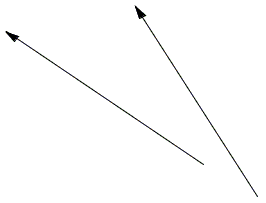# What Is Line?

Line (actually a straight line), together with point, is a basic concept of elementary geometry. The idea of line is an abstraction that distills our intuition that a straight line is the shortest way between two points. However, we distinguish between a line and a line segment. A line segment includes the endpoints, i.e. the points that it joins. The line through the two points continues beyond these points indefinitely.

The absence of the endpoints is often hinted to by drawing arrows:In the (Euclidean) plane lines are subject to a dichotomy: two distinct lines either intersect or are parallel. Two lines that intersect define a unique point - the point of intersection. The point of intersection lies on (or belongs to) both lines. Parallel lines have no common points.

Every point on a line divides the line into two parts. These are called rays. A ray is a piece of a line with one endpoint. (Rays are used in defining angles.)

Rays and line segments are said to be parallel if the lines they are parts of are parallel. Rays and line segments are not subject to the dichotomy. Not parallel rays or line segments need not intersect:A parallelogram is a quadrilateral with sides on two pairs of parallel lines.•